3.2.3 Kinetic Model of an Ideal Gas

Kinetic Model of an Ideal Gas

Gas Pressure

• A gas is made of a large number of particles
• Gas particles have mass and move randomly at high speeds
• Pressure in a gas is due to the collissions of the gas particles with the walls of the container that holds the gas
• When a gas particle hits a wall of the container, it undergoes a change in momentum due to the force exerted by the wall on the particle (as stated by Nerwton's second law)
• According to Newton's third law, there is an equal and opposite force exerted by the particle on the wall
• Since there is a large number of particles, their collisions with the walls of the container give rise to gas pressure, which is calculated as follows: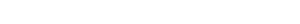• Where:
• p = pressure in pascals (Pa)
• F = force in newtons (N)
• A = area in metres squared (m2)

Average Random Kinetic Energy of Gas Particles

• Particles in gases have a variety of different speeds
• The absolute temperature of a gas is directly proportional to the average random kinetic energy of the particles EK, which can be written as follows: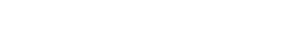• Where:
• EK = average random kinetic energy of the particles in joules (J)
• kB = 1.38 × 10–23 J K–1
• T = absolute temperature in kelvin (K)
• kB is known as Boltzmann’s constant, and it can be written as follows: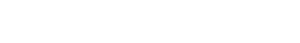• Where:
• R = 8.31 J K–1 mol–1  (ideal gas constant)
• NA = 6.02 × 1023 mol–1 (Avogadro constant)

Internal Energy of the Gas

• Since the intermolecular forces between the particles in a gas are negligible, the internal energy U of the gas is equal to the total kinetic energy of the gas particles:

U = NEK

• Where:
• U = internal energy of the gas in joules (J)
• N = number of particles
• EK = average random kinetic energy of the particles in joules (J)
• Using the relationship between number of particles N and moles n, and the expression of Boltzmann’s constant in terms of the ideal gas and Avogadro constants (R and NA respectively), the above equation can be rewritten as follows: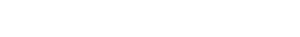• Where:
• U = internal energy of the gas in joules (J)
• n = number of moles in the gas (mol)
• R = 8.31 J K–1 mol–1  (ideal gas constant)
• T = absolute temperature in kelvin (K)
• Alternatively, using the equation of state of ideal gases, the internal energy can also be written as follows: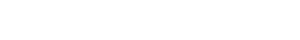• Where:
• U = internal energy of the gas in joules (J)
• p = gas pressure in pascals (Pa)
• V = gas volume in metres cubed (m3)

Worked Example

2 mol of gas is sealed in a container, at a temperature of 47°C.

Determine:

• The average random kinetic energy of the particles in the gas
• The internal energy of the gas

Step 1: Write down the temperature T of the gas in kelvin (K)

T = 47°C = 320 K

Step 2: Write down the equation linking the absolute temperature T of the gas to the average random kinetic energy EK of the gas particlesStep 3: Substitute numbers into the equation

• From the data booklet, kB = 1.38 × 10–23 J K–1EK = 6.6 × 10–21 J

Step 4: Write down the equation linking the internal energy U of the gas to the number of moles n and the absolute temperature TStep 5: Substitute numbers into the equation

• From the data booklet, R = 8.31 J K–1 mol–1U = 8000 J = 8kJ

Note that, alternatively, the internal energy can be calculated using the following equation:

U = NEK = 8000 J = 8kJ

• N = nNA = 2 mol × (6.02 × 1023) mol–1 = 1.2 × 1024
• EK = 6.6 × 10–21 J (calculated in Step 3)

Real & Ideal Gases

• The equation of state of ideal gases and the equations for the kinetic energy of the particles and the internal energy of the gas derived previously, only apply to ideal gases
• An ideal gas is defined as one to which all the statements below apply:
• It contains a very large number of identical particles (atoms or molecules)
• Each gas particle occupies a negligible volume compared to the volume of the gas (i.e. they can be considered as point particles)
• The gas particles move randomly at high speeds
• The gas particles obey Newton’s laws of motion
• There are no intermolecular forces between the gas particles, therefore the internal energy of the gas is equal to the total kinetic energy of the particles (U = NEK)
• The gas particles undergo elastic collisions with each other and with the walls of the container in which the gas is held, hence the total kinetic energy of the particles and the gas temperature do not change as a result of these collisions
• The duration of the collisions is negligible compared with the time interval between collisions
• Each particle exerts a force on the wall of the container with which it collides; the average of the forces produced by all gas particles gives rise to uniform gas pressure
• Real gases are not ideal gases, however, under certain conditions, they can be considered as ideal gases. An ideal gas is a good approximation of a real gas when:
• Pressure and density are not too high
• Temperature is not too low
CloseClose